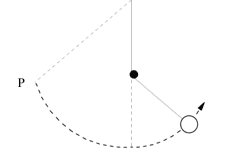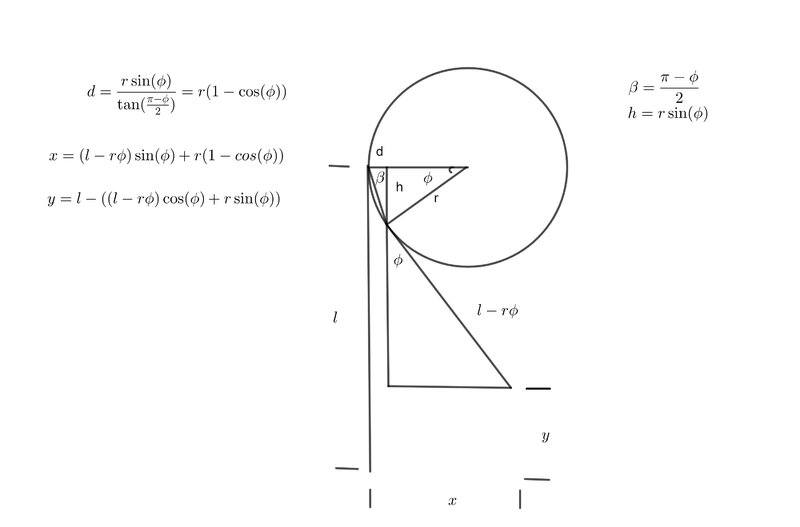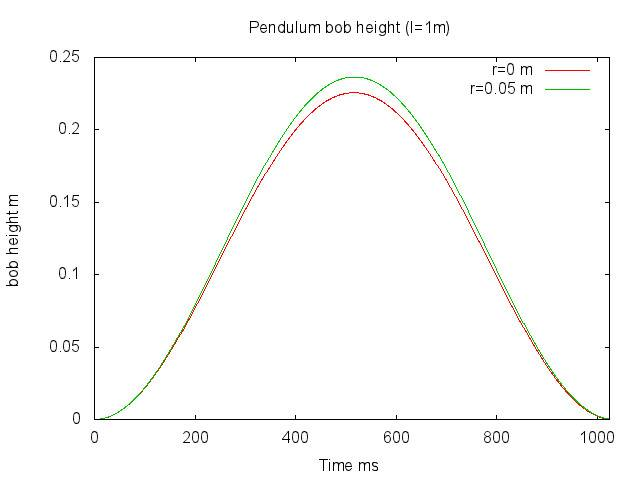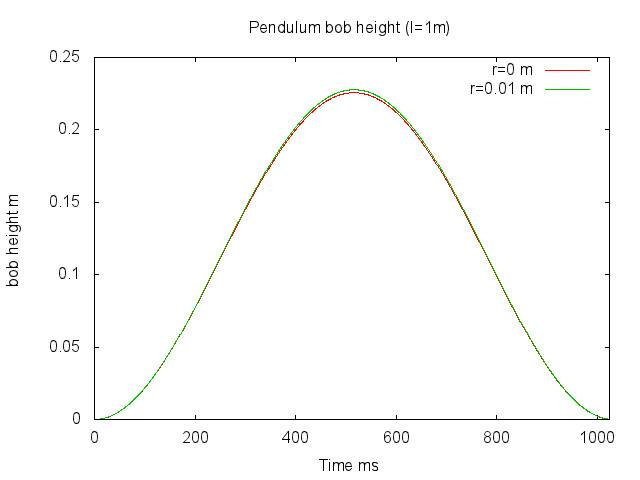# Height of pendulum after hitting a peg

• songoku

#### songoku

Homework Statement
A pendulum bob is released from point P. As it swings down, the string strikes a peg and the bob swings up in a different arc on the other side as shown (please see below). The bob will swing
A. up to a lesser height than P.
B. up to the same height as P.
C. up to a greater height than P.
D. round and round the peg
Relevant Equations
Conservation of energyBy considering conservation of energy, my answer is (B). But the solution states the answer is (A)

Is it because there will be loss of some of the KE when the string hits the peg so making answer (A) is better than answer (B)? The question itself does not state about friction

Thanks

•Delta2

•songoku
Angular momentum = mvr

Since the radius of motion decreases, the velocity will increase. So the correct answer should be (C)?

Thanks

•PeroK
You perhaps need to think a little bit more than that!

•songoku
Me too.
The only way it could lower is if it were to reach max height with some remaining horizontal velocity, but that would require the release height to be above the peg. It is clearly not.

•songoku

I don't see how that will produce a different answer.

If the peg is of infinitesimal radius I think the answer is (B).

I don't see how that will produce a different answer.
I guess if everything is idealised, there is theoretically no way to lose energy. But, practically, the collision with the peg must cost something.

One of my favorite lab practicum exercise when I used to teach was based on this interrupted pendulum. Students had to figure out the original height of release so that after hitting the peg, the pendulum bob would loop-the-loop, and then collide with a second peg above and to the right of the first peg (ref. photo in post #8). The second peg was placed so that the string had to go slack for the collision to occur. Students were given a meter stick and 3 attempts to make it work. I liked this exercise because it brought together three basic areas in mechanics, energy conservation, free body diagrams and projectile motion.

•songoku and PeroK
If the peg is of infinitesimal radius I think the answer is (B).
Again, I don’t see what that has to with it unless the peg is so thick that the string goes above the horizontal on the way up.
practically, the collision with the peg must cost something.
So consider air resistance too, then we do not even need the peg.
@songoku , where is this solution coming from? Is it a book? Online?

So consider air resistance too, then we do not even need the peg.
You can see in the experiment that the height lost on a normal swing of the pendulum is negligible but that the pendulum loses a small but observable amount of height every time it hits the peg.

Yeah you are correct. In my (albeit weak) defense I note that what I said was in fact correct...just slightly restrictive ! I'll stop now.

You can see in the experiment that the height lost on a normal swing of the pendulum is negligible but that the pendulum loses a small but observable amount of height every time it hits the peg.
I've watched it several times and cannot discern any greater loss in the right to left swing than in the left to right.
There is another subtle reason why it might reach a lower peak on the left side than on the right. The increased tension in the tighter arc could leave some energy stored as elastic energy in the section above the peg, friction preventing its being released until the next swing to the right.
But I cannot believe the question would intend us to clutch any of these straws without more of a clue. The answer is just wrong.

•songoku and berkeman
I've watched it several times and cannot discern any greater loss in the right to left swing than in the left to right.
There is another subtle reason why it might reach a lower peak on the left side than on the right. The increased tension in the tighter arc could leave some energy stored as elastic energy in the section above the peg, friction preventing its being released until the next swing to the right.
But I cannot believe the question would intend us to clutch any of these straws without more of a clue. The answer is just wrong.
When the string comes in contact with the peg the subsystem arm is shortened by ##r\phi## where ##r## is the radius of the peg and ##\phi## is the angle measuring how much the string raps around the peg. This shortening of the subsystem arm causes it to increase in frequency. By conservation of energy the increasing frequency must be compensated by a decrease in amplitude.

•Delta2
By conservation of energy the increasing frequency must be compensated by a decrease in amplitude.
That does not follow. If I reduce the mass on a spring, same amplitude, the frequency goes up but the energy does not.
In the present context, the energy is directly related to the height reached each side, so if no energy is lost they must be the same.

Last edited:
When the string comes in contact with the peg the subsystem arm is shortened by ##r\phi## where ##r## is the radius of the peg and ##\phi## is the angle measuring how much the string raps around the peg. This shortening of the subsystem arm causes it to increase in frequency. By conservation of energy the increasing frequency must be compensated by a decrease in amplitude.
Sorry, where does the energy come from to change the GPE?

EDIT -- beaten out by @haruspex

I've watched it several times and cannot discern any greater loss in the right to left swing than in the left to right.
There is another subtle reason why it might reach a lower peak on the left side than on the right. The increased tension in the tighter arc could leave some energy stored as elastic energy in the section above the peg, friction preventing its being released until the next swing to the right.
But I cannot believe the question would intend us to clutch any of these straws without more of a clue. The answer is just wrong.
Also, if the string has non-negligible mass, there is a discontinuity of its velocity, both the part below the peg along the string length which has a different angular velocity, peg and no peg states, and above the peg where the string abruptly decelerates to zero angular velocity on hitting the peg, and accelerates abruptly when being removed from the peg.

The bob has the same kinetic energy at the bottom of the swing, so in the really ideal situation PE and KE exchange would be lossless, so B would be the answer.

For the real lossy situation, A would be the answer.

The OP doesn't give which case to consider, but I would pick B due to the question being about conservation of energy. In fact, if there was no peg at all, the most obvious answer would be B, if no losses are accounted for. Why not assume the same for the peg situation.

Not a fair test question.

•songoku and PeroK
You perhaps need to think a little bit more than that!
Sorry I still don't understand. The angular momentum will be conserved since no net external torque acting on the object. Am I correct up until this part?

The only way it could lower is if it were to reach max height with some remaining horizontal velocity, but that would require the release height to be above the peg. It is clearly not.
Sorry I also don't understand this part. If I assume there is no friction, won't the pendulum will also reach the same height as the point of release? How can the pendulum reach max height with some remaining horizontal velocity? Won't the velocity be zero when it reaches max height so it can gain velocity in opposite direction and swing back?
@songoku , where is this solution coming from? Is it a book? Online?
Book. Only final answer without explanation

Thanks

Won't the velocity be zero when it reaches max height
Not if the energy is enough to get it above the peg.
Book
Which book?

•songoku
Not if the energy is enough to get it above the peg.
I am not sure how to imagine the motion. Will the motion of the bob become something like projectile motion when it goes above the peg and reaching maximum height with some remaining horizontal velocity?

Which book?
Sorry I don't know the book. The teacher just sent photos of questions and solutions from the book to students

Will the motion of the bob become something like projectile motion when it goes above the peg and reaching maximum height with some remaining horizontal velocity?
It depends. If it started high enough it will have so much speed that the string will remain taut as it wraps around the peg. Less than that, but enough to rise above the peg, the string will go slack and the bob move as a projectile for a while.
The teacher just sent photos of questions and solutions from the book
What does the photo of the answer consist of? Just a letter A? An A preceded by the question number to match it up with the question photo? Some explanation provided?

•songoku
What does the photo of the answer consist of? Just a letter A? An A preceded by the question number to match it up with the question photo? Some explanation provided?That's part of the answer key. Question number matches answer key number. No explanation at all

Thank you very much for all the help and explanation PeroK, haruspex, hutchphd, kuruman, Fred Wright, berkeman, 256bits

•berkeman
Could be a misprint that escaped the proofreader.

•songoku and Delta2
That does not follow. If I reduce the mass on a spring, same amplitude, the frequency goes up but the energy does not.
In the present context, the energy is directly related to the height reached each side, so if no energy is lost they must be the same.
You are absolutely right! I was guessing and when I guess I usually make myself the fool. I, however, determined to get to the bottom of this controversy, so I analyzed the subsystem using Lagrangian dynamics. I derived the geometry of the situation in this figure:From this I came up with equation of motion for the subsystem:
$$\ddot \phi = \frac{2r\dot{ \phi}^2}{l-r\phi}-\frac{g\sin{\phi}}{l-r\phi}$$
I wrote a C program to integrate this equation using the Runge-Kutta (RK4) algorithm (neglecting air resistance). The first plot below shows the height of the bob vs time with ##r=5cm## and ##l=1 m## compared to the height with ##r=0##.The analysis shows that the height exceeds the ##r=0## condition. Below is a plot where ##r=1cm##.Clearly, as the radius of the peg becomes smaller, the difference in height becomes negligible. I don't blame you for observing my analysis with a jaundiced eye, but wanted to get a handle on the situation and go beyond my silly guess.